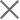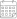Subscribe

### Indexes

April 2022 | Electricity Market Review27 May 2022

In April 2022, Georgian power plants generated 1,125 mln. kWh of electricity (Figure 1). This represents a 36% increase in total generation, compared to the previous year (in April 2021, the total generation was 825 mln. kWh). The increase in generation on a yearly basis comes from the increase of 44% in hydro power generation, respectively, more than offsetting the 46% and 6% decline in thermal power and wind power generation, respectively.

On a monthly basis, generation increased by approximately 4% (in March 2022, total generation was 1077 mln. kWh) (Figure 1). The monthly increase in total generation is induced by a 90% increase in hydropower generation, while thermal power and wind power generation fell by 93%, and 9%, respectively.

The consumption of electricity on the local market was 1156 mln. kWh (+10% compared to April 2021, and -14% compared to March 2022) (Figure 1). In April 2022, power consumption exceeded generation by 30 mln. kWh which was 3% of total generation (in April 2021 difference between total generation and consumption resulted in a deficit of 224 mln. kWh, around 27% of the total generation for the month).